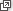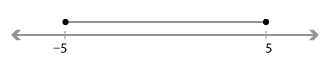## Content

### Set theory

It is not possible to discuss functions sensibly without using the language and ideas of elementary set theory. In particular, we will use the following ideas from the module Sets and Venn diagrams(Years 7–8):

• sets and their elements
• equal sets
• listing the members of a set; for example, $$A = \{2,4,6,8\}$$
• set membership; for example, $$4\in A$$ and $$5\notin A$$
• finite and infinite sets, and the number of elements in a finite set
• subsets
• unions and intersections.

Sets must be well defined, and if sets are defined using mathematical notation there are rarely any problems. A set is well defined if it is possible to determine whether or not a given object belongs to that set or not. The set of all words in the English language is not well defined.

##### Exercise 1

Think of several reasons why 'the set of all English words' is not well defined.

#### Further notation

Set-builder notation is useful for describing subsets of the real numbers. For example, consider the set defined by

$S = \{\, x\in \mathbb{R} \mid -5\leq x\leq 5 \,\}$

or, equivalently,

$S = \{\, x\in \mathbb{R} : -5\leq x\leq 5 \,\}.$

This can be read as '$$S$$ is the set of all $$x$$ belonging to $$\mathbb{R}$$ such that $$-5\leq x\leq 5$$'. In this construction:

• $$S$$ is the name of the set
• $$\{\quad\}$$ holds the definition together
• $$x\in \mathbb{R}$$ says that $$x$$ is a real number
• both $$\mid$$ and $$:$$ mean 'with the property that'
• $$-5\leq x\leq 5$$ limits the allowed values of $$x$$.

So $$S$$ is the following interval:If we define the set $$T = \{\, x\in \mathbb{R} : |x|\leq 5 \,\}$$, then $$S = T$$.

Union and intersection are familiar operations on sets. Another useful operation on sets is set difference. For two sets $$A$$ and $$B$$, we define

$A \setminus B = \{\, x \in A \mid x \notin B \,\}.$

That is, $$A \setminus B$$ is the set of all elements of $$A$$ that are not in $$B$$. We can read $$A \setminus B$$ as '$$A$$ take away $$B$$' or '$$A$$ minus $$B$$'. For example, the set of all non-zero real numbers can be written as $$\{\, x \in \mathbb{R} \mid x \neq 0 \,\}$$ or, more simply, as $$\mathbb{R} \setminus \{0\}$$.

The Cartesian product of two sets $$A$$ and $$B$$ is defined by

$A\times B = \{\, (a,b) \mid a\in A \text{ and } b\in B \,\}.$

That is, $$A\times B$$ is the set of all ordered pairs $$(a,b)$$ with $$a$$ in $$A$$ and $$b$$ in $$B$$. So, for example,

$\mathbb{R}\times\mathbb{R} = \{\, (x,y) \mid x\in \mathbb{R} \text{ and } y\in \mathbb{R} \,\}.$

We write $$\mathbb{R}\times\mathbb{R}$$ as $$\mathbb{R}^2$$, which explains the use of the notation $$\mathbb{R}^2$$ to describe the coordinate plane. This was introduced in the module Coordinate geometry .

Note. In this module and many others:
• $$\mathbb{R}$$ denotes the set of real numbers
• $$\mathbb{Q}$$ denotes the set of rational numbers
• $$\mathbb{Z}$$ denotes the set of integers
• $$\mathbb{C}$$ denotes the set of complex numbers.

Next page - Content - Set theory